Calculus 2 : Introduction to Integrals

Example Questions

1 2 10 11 12 13 14 15 16 18 Next →

Example Question #23 : Fundamental Theorem Of Calculus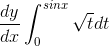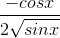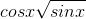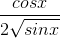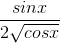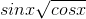Explanation:

This is a Second Fundamental Theorem of Calculus problem.  Since the derivative cancels out the integral, we just need to plug in the bounds into the function (top bound - bottom bottom) and multiply each by their derivative.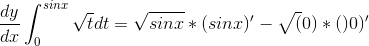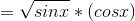.

Example Question #24 : Fundamental Theorem Of Calculus

Differentiate: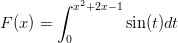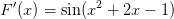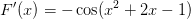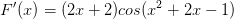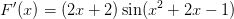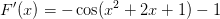Explanation:

Differentiate:Use the Second Fundamental Theorem of Calculus:

________________________________________________________

If the function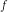is continuous on an open interval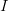and ifis in, then for a function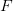defined by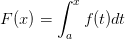, we have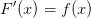________________________________________________________________

To understand how to apply the second FTOC, notice that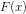in our case is a function of a function. The chainrule will therefore have to be applied as well. To see how this works, let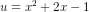. The function can now be written in the form: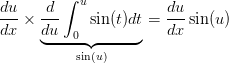Now we can go back to writing in terms of, the derivative is,Example Question #25 : Fundamental Theorem Of Calculus

In exponentially decaying systems, often times the solutions to differential equations take on the form of an integral called Duhamel's Integral. This is given by: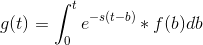Whereis a constant and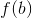is a function that represents an external force.

Equations of the form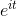are  sinusoidal functions, whereis the imaginary constant. Determine the external forceneeded to produce solutions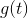of sinusoidal behavior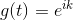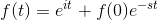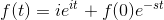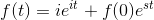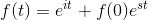Explanation:

We plug in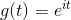into our formula and determine the external force.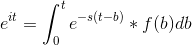Taking the derivative of each side with respect to, and applying the fundamental theorem of calculus: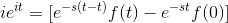Solving for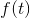,Example Question #26 : Fundamental Theorem Of Calculus

In harmonic systems, often times the solutions to differential equations take on the form of an integral called Duhamel's Integral. This is given by:Whereis a constant andis a function that represents an external force.

What external force is needed in order to obtain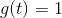if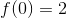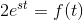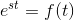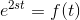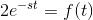Explanation:

In order to solve this, we substituteinto our equation and solve for: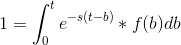Taking the derivative of each side with respect to, we get that: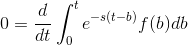Using the fundamental theorem of calculus: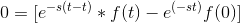Solving for: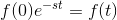Since,Example Question #27 : Fundamental Theorem Of Calculus

Let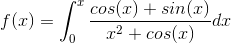Find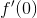.Explanation:

The Fundamental Theorem of Calculus tells us that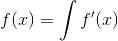therefore,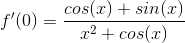From here plug in 0 into this equation.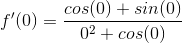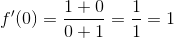1 2 10 11 12 13 14 15 16 18 Next →